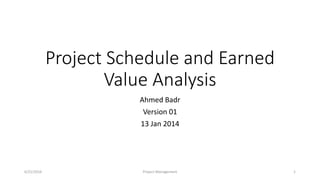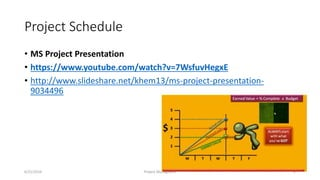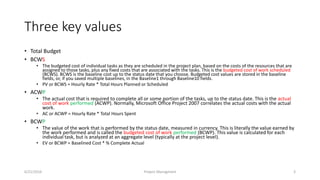Se está descargando tu SlideShare. ×
Anuncio
Anuncio
Anuncio
Anuncio
Anuncio
Anuncio
Anuncio
Anuncio
Anuncio
Anuncio
AnuncioCargando en…3
×

1 de 21 Anuncio

# Earned value 01

show you how to use MS project to apply Earned Value method to track project performance from time to cost

show you how to use MS project to apply Earned Value method to track project performance from time to cost

Anuncio
Anuncio

### Earned value 01

1. 1. Project Schedule and Earned Value Analysis Ahmed Badr Version 01 13 Jan 2014 6/21/2016 Project Management 1
2. 2. Project Schedule • MS Project Presentation • https://www.youtube.com/watch?v=7WsfuvHegxE • http://www.slideshare.net/khem13/ms-project-presentation- 9034496 6/21/2016 Project Managment 2
3. 3. Three key values • Total Budget • BCWS • The budgeted cost of individual tasks as they are scheduled in the project plan, based on the costs of the resources that are assigned to those tasks, plus any fixed costs that are associated with the tasks. This is the budgeted cost of work scheduled (BCWS). BCWS is the baseline cost up to the status date that you choose. Budgeted cost values are stored in the baseline fields, or, if you saved multiple baselines, in the Baseline1 through Baseline10 fields. • PV or BCWS = Hourly Rate * Total Hours Planned or Scheduled • ACWP • The actual cost that is required to complete all or some portion of the tasks, up to the status date. This is the actual cost of work performed (ACWP). Normally, Microsoft Office Project 2007 correlates the actual costs with the actual work. • AC or ACWP = Hourly Rate * Total Hours Spent • BCWP • The value of the work that is performed by the status date, measured in currency. This is literally the value earned by the work performed and is called the budgeted cost of work performed (BCWP). This value is calculated for each individual task, but is analyzed at an aggregate level (typically at the project level). • EV or BCWP = Baselined Cost * % Complete Actual 6/21/2016 Project Managment 3
4. 4. Cost Variance % • Cost Variance (CV) = Earned Value (EV) - Actual Cost (AC) • Or • Cost Variance (CV) = BCWP - ACWP Cost Variance • Cost Variance % indicates how much over or under budget the project is in terms of percentage. • CV % = Cost Variance (CV) / Earned Value (EV) • Or • CV % = CV / BCWP 6/21/2016 Project Managment 4
5. 5. Cost Performance Indicator (CPI) • Cost Performance Indicator is an index showing the efficiency of the utilization of the resources on the project. Cost Performance Indicator can be calculated using the following formula: • CPI = Earned Value (EV) / Actual Cost (AC) • Or • CPI = BCWP / ACWP 6/21/2016 Project Managment 5
6. 6. Schedule Variance • Schedule Variance indicates how much ahead or behind schedule the project is. • Schedule Variance (SV) = Earned Value (EV) - Planned Value (PV) • Or • Schedule Variance (SV) = BCWP - BCWS Schedule Variance % • Schedule Variance % indicates how much ahead or behind schedule the project is in terms of percentage. • SV % = Schedule Variance (SV) / Planned Value (PV) • Or • SV % = SV / BCWS 6/21/2016 Project Managment 6
7. 7. Schedule Performance Indicator (SPI) • Schedule Performance Indicator is an index showing the efficiency of the time utilized on the project. Schedule Performance Indicator can be calculated using the following formula: • SPI = Earned Value (EV) / Planned Value (PV) • Or • SPI = BCWP / BCWS 6/21/2016 Project Managment 7
8. 8. To Complete Schedule Performance Indicator (TSPI) • To Complete Schedule Performance Indicator is an index showing the efficiency at which the remaining time on the project should be utilized. This can be calculated using the following formula: • TSPI = ( Total Budget - EV ) / ( Total Budget - PV ) • Or • TSPI = ( Total Budget - BCWP ) / ( Total Budget - BCWS ) 6/21/2016 Project Managment 8
9. 9. MS Project - Earned Value • Tables • Entry – Resources Sheet - Cost • On the View menu, point to Table, and then click More Tables • In the Tables list, click Earned Value, Earned Value Cost Indicators, or Earned Value Schedule Indicators, and then click Apply. • Create Baseline • Set “advanced” options to calculate based in which baseline • And set the Earned Value Calculation method • Reports • Use the Earned Value Over Time report to view a chart that plots AC (actual cost of work performed), planned value (budgeted cost of work scheduled), and earned value (budgeted cost of work performed) over time. To generate this report, on the Report menu, click Visual Reports. Click Earned Value Over Time and then click View 6/21/2016 Project Managment 9
10. 10. 6/21/2016 Project Managment 10
11. 11. Plan-Track-Analyze 6/21/2016 Project Managment 11
12. 12. 6/21/2016 Project Managment 12
13. 13. 6/21/2016 Project Managment 13
14. 14. Earned Value over Time Report 6/21/2016 Project Managment 14
15. 15. Project Forecast • Budget At Completion (BAC) • Budget At Completion (BAC) is the total budget allocated to the project. • Budget At Completion (BAC) is generally plotted over time. Say like periods of reporting (Monthly, Weekly, etc.) • BAC is used to compute the Estimate At Completion (EAC), explained in next section. • BAC is also used to compute the TCPI and TSPI. • BAC = Baseline Effort-hours * Hourly Rate 6/21/2016 Project Managment 15
16. 16. Estimate To Complete (ETC) • Estimate To Complete (ETC) is the estimated cost required to complete the remainder of the project. • Estimate To Complete (ETC) is calculated and applied when the past estimating assumptions become invalid and a need for fresh estimates arises. • ETC is used to compute the Estimation At Completion (EAC). 6/21/2016 Project Managment 16
17. 17. Estimate At Completion (EAC) • Estimate At Completion (EAC) is the estimated cost of the project at the end of the project. • There are three methods to calculate EAC: • Variances are Typical - This method is used when the variances at the current stage are typical and are not expected to occur in the future. • Past Estimating Assumptions are not valid - This method is used when the past estimating assumptions are not valid and fresh estimates are applied to the project. • Variances will be present in the future - This method is used when the assumption is that the current variances will continue to be present in the future. • The formulas for calculation of the three methods are as given below: • AC + (BAC - EV) • AC + ETC (Estimate To Complete) • AC + (BAC- EV) / CPI 6/21/2016 Project Managment 17
18. 18. Variance At Completion (VAC) • Variance At completion (VAC) is the variance on the total budget at the end of the project. • This is the difference between what the project was originally expected (baselined) to cost, versus what the it is now expected to cost. • VAC = BAC – EAC • % Completed Planned • The percentage of work which was planned to be completed by the Reporting Date. This is calculated using the following formula: • % Completed Planned = PV / BAC • % Completed Actual • The percentage of work which was actually completed by the Reporting Date. This is calculated using the following formula: • % Completed Actual = AC / EAC 6/21/2016 Project Managment 18
19. 19. Earned Value Focuses on • % Completed Planned • The percentage of work which was planned to be completed by the Reporting Date. This is calculated using the following formula: • % Completed Planned = PV / BAC • % Completed Actual • The percentage of work which was actually completed by the Reporting Date. This is calculated using the following formula: • % Completed Actual = AC / EAC 6/21/2016 Project Managment 19
20. 20. Plan-Track-Analyze-Forecast 6/21/2016 Project Managment 20
21. 21. Forecast after Change Status Day 6/21/2016 Project Managment 21

### Notas del editor

• http://www.tutorialspoint.com/earn_value_management/cost_variance.htm
• The formula mentioned above gives the efficiency of the project team in utilizing the time allocated for the project.
SPI value above 1 indicates project team is very efficient in utilizing the time allocated to the project.
SPI value below 1 indicates project team is less efficient in utilizing the time allocated to the project.
• https://support.office.com/en-us/article/Analyze-project-performance-with-earned-value-analysis-29efe3a3-dc4d-4e3f-833b-08c3b4599a04
• EVM (Earn Value Management) and MS PROJECT 2010
http://ntrajkovski.com/2012/04/06/evm-earn-value-management-and-ms-project-2010/
• For the second way of calculating the EAC, assumed I needed 500 LE to complete the project